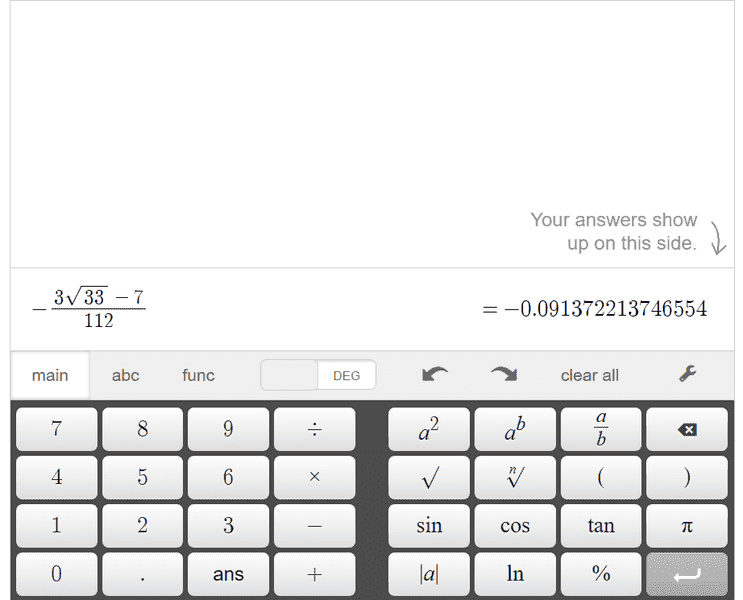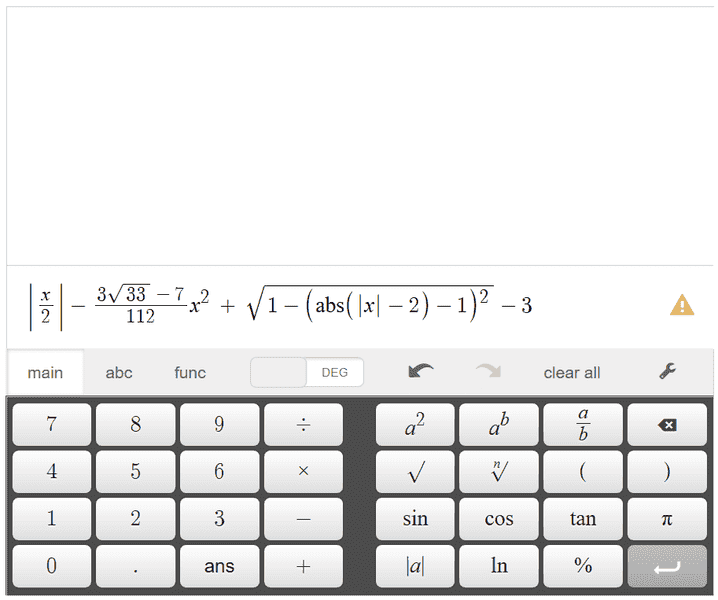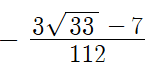# Why to write numbers in square roots and not in decimals?

• B
• pairofstrings

#### pairofstrings

Hi. I have coefficient of x2 asin an expression that looks like this* calculator shows little yellow triangle because 'x' is not defined.

If I can write the coefficient of x2 as - 0.091372213746554 then why did the author write coefficient of x2 like this shown below?Thanks.

#### Attachments

Last edited:
the author
Of what?

-0.091372213746554 is not exact. It is only an approximation, where the number of digits is determined by the the calculator. A better approximation is:
-0.091372213746554339103141378613 - but that is again not exact, it just has a smaller error. No matter how many digits you add you always have an error.

##\displaystyle -\frac{3\sqrt{33}-4}{112}## is exact.

•symbolipoint and jedishrfu
@pairofstrings, you seem to have a fundamental misunderstanding of the difference between numbers represented by radicals and decimal approximations of them. For example, the exact value of the diagonal of a square 1 unit on each side is ##\sqrt 2##. This value can be approximated by 1.414 or 1.4142 or 1.41421 or even 1.4142135623730950488016887242097, but none of them is exactly equal to ##\sqrt 2##.

##\sqrt 2## is an example of an irrational number, one whose decimal represention takes an infinite number of digits to the right of the decimal point, with no repeating pattern.